Atmos. Chem. Phys., 18, 7799–7814, 2018
https://doi.org/10.5194/acp-18-7799-2018
Atmos. Chem. Phys., 18, 7799–7814, 2018
https://doi.org/10.5194/acp-18-7799-2018

Research article 04 Jun 2018

Research article | 04 Jun 2018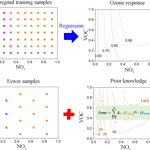# Quantification of the enhanced effectiveness of NOx control from simultaneous reductions of VOC and NH3 for reducing air pollution in the Beijing–Tianjin–Hebei region, China

Quantification of the enhanced effectiveness of NOx control from simultaneous reductions of VOC and NH3 for reducing air pollution in the Beijing–Tianjin–Hebei region, China
Jia Xing1,2, Dian Ding1, Shuxiao Wang1,2, Bin Zhao1,2,5, Carey Jang3, Wenjing Wu1, Fenfen Zhang1, Yun Zhu4, and Jiming Hao1,2 Jia Xing et al.
• 1State Key Joint Laboratory of Environmental Simulation and Pollution Control, School of Environment, Tsinghua University, Beijing 100084, China
• 2State Environmental Protection Key Laboratory of Sources and Control of Air Pollution Complex, Beijing 100084, China
• 3The U.S. Environmental Protection Agency, Research Triangle Park, NC 27711, USA
• 4College of Environmental Science & Engineering, South China University of Technology, Guangzhou Higher Education Mega Center, Guangzhou, China
• 5Joint Institute for Regional Earth System Science and Engineering and Department of Atmospheric and Oceanic Sciences, University of California, Los Angeles, CA 90095, USA

Correspondence: Shuxiao Wang (shxwang@tsinghua.edu.cn)

Abstract

As one common precursor for both PM2.5 and O3 pollution, NOx gains great attention because its controls can be beneficial for reducing both PM2.5 and O3. However, the effectiveness of NOx controls for reducing PM2.5 and O3 are largely influenced by the ambient levels of NH3 and VOC, exhibiting strong nonlinearities characterized as NH3-limited/NH3-poor and NOx-/VOC-limited conditions, respectively. Quantification of such nonlinearities is a prerequisite for making suitable policy decisions but limitations of existing methods were recognized. In this study, a new method was developed by fitting multiple simulations of a chemical transport model (i.e., Community Multiscale Air Quality Modeling System, CMAQ) with a set of polynomial functions (denoted as “pf-RSM”) to quantify responses of ambient PM2.5 and O3 concentrations to changes in precursor emissions. The accuracy of the pf-RSM is carefully examined to meet the criteria of a mean normalized error within 2 % and a maximal normalized error within 10 % by using 40 training samples with marginal processing. An advantage of the pf-RSM method is that the nonlinearity in PM2.5 and O3 responses to precursor emission changes can be characterized by quantitative indicators, including (1) a peak ratio (denoted as PR) representing VOC-limited or NOx-limited conditions, (2) a suggested ratio of VOC reduction to NOx reduction to avoid increasing O3 under VOC-limited conditions, (3) a flex ratio (denoted as FR) representing NH3-poor or NH3-rich conditions, and (4) enhanced benefits in PM2.5 reductions from simultaneous reduction of NH3 with the same reduction rate of NOx. A case study in the Beijing–Tianjin–Hebei region suggested that most urban areas present strong VOC-limited conditions with a PR from 0.4 to 0.8 in July, implying that the NOx emission reduction rate needs to be greater than 20–60 % to pass the transition from VOC-limited to NOx-limited conditions. A simultaneous VOC control (the ratio of VOC reduction to NOx reduction is about 0.5–1.2) can avoid increasing O3 during the transition. For PM2.5, most urban areas present strong NH3-rich conditions with a PR from 0.75 to 0.95, implying that NH3 is sufficiently abundant to neutralize extra nitric acid produced by an additional 5–35 % of NOx emissions. Enhanced benefits in PM2.5 reductions from simultaneous reduction of NH3 were estimated to be 0.04–0.15 µg m−3 PM2.5 per 1 % reduction of NH3 along with NOx, with greater benefits in July when the NH3-rich conditions are not as strong as in January. Thus, the newly developed pf-RSM model has successfully quantified the enhanced effectiveness of NOx control, and simultaneous reduction of VOC and NH3 with NOx can assure the control effectiveness of PM2.5 and O3.

Share
1 Introduction

Tropospheric ozone (O3) and fine particulate matter (PM2.5) are two major air pollutants that exert significant effects on human health (Forouzanfar et al., 2015; GBD-MAPS, 2016; Cohen et al., 2017) and the global climate (Myhre et al., 2013). Effective controls on the anthropogenic sources of O3 and PM2.5 are necessary to reduce their harmful effects on health and climate. As one common precursor for both O3 and PM2.5, NOx significantly influences the ambient concentrations of O3 and PM2.5. Previous studies suggested that the deterioration of air quality in China over past 2 decades is highly associated with the increasing trend of national NOx emissions (Wang et al., 2011), which are estimated to increase from 11.0 Mt in 1995 to 26.1 Mt in 2010 (Zhao et al., 2013). Since the early 2010s (late 2000s in some regions such as Pearl River Delta), strict regulations have been implemented on power plants and vehicle emissions, leading to a considerable NO2 reduction witnessed by the declining trend in satellite-retrieved NO2 column densities (i.e., reduced by 32 % from 2011 to 2015; Liu et al., 2016). However, the reduction in PM2.5 is not as significant as that in NO2 or SO2 (Fu et al., 2017). The reason might be associated with the increases in NH3, which has not been well controlled to date in China and exhibits an increasing trend of nearly 20 % from 2011 to 2014 observed from satellite retrievals (Fu et al., 2017). Such increases in NH3 weakened the control effectiveness of SO2 and NO2 in PM2.5 reduction (Wang et al., 2011; Fu et al., 2017). Worse still, recently O3 concentrations have exhibited an increasing trend in some cities in the Yangtze River Delta and Pearl River Delta (Li et al., 2014). The number of days on which O3 concentration exceeded the national standard (i.e., 8 h maxima level less than 160 µg m−3) increased from 7.2 % in 2010 to 12.7 % in 2015 in Shanghai. The annual averaged O3 increased by 0.86 ppb yr−1 from 2006 to 2011 in Guangdong, accompanied by a corresponding NO2 reduction of 0.61 ppb yr−1 (Li et al., 2014). The recent observation data suggested a continued increasing trend of 8 h maxima O3 in Zhuhai (from 128 to 142 µg m−3) and Shenzhen (from 122 to 134 µg m−3) in the Pearl River Delta from 2013 to 2016. Such an increase in O3 is likely to be associated with the NOx reductions in the area that are located in the volatile organic compound (VOC)-limited conditions (i.e., decreased NOx leads to increased O3), implying the disbenefit of NOx controls for O3 reduction under VOC-limited conditions. How to assure the effectiveness of NOx controls for reducing O3 and PM2.5 becomes a difficult challenge for policy design (Cohan et al., 2005; Tsimpidi et al., 2008).

To address that challenge, studies on investigating the relationship among the responses of O3 and PM2.5 to precursor emission changes have been conducted. Indicators such as NOy, H2O2HNO3 and H2O2 (O3+ NO2) as well as the degree of sulfate neutralization, gas ratio and adjusted gas ratio are used to define the O3 and PM2.5 chemistry in many studies (Sillman et al., 1995; Tonnesen et al., 2000; Zhang et al., 2009; Liu et al., 2010; Ye et al., 2016). The aforementioned indicators can provide rapid assumptions for the baseline status of pollution sensitivities to precursor emissions. Modeling studies with chemistry–transport models (CTMs) have been conducted to investigate the responses of O3 and PM2.5 to emission perturbation through sensitivity analyses, such as decoupled direct methods (DDMs) and high-order DDMs (Hakami et al., 2003; Cohan et al., 2005), and source apportionment technology such as ozone source apportionment technology (Dunker et al., 2002), particulate matter source apportioning technology (Wagstrom et al., 2008) and the integrated source apportionment method (Kwok et al., 2013, 2015). A statistical response surface model (RSM) has been developed and successfully used in O3 and PM2.5 response simulations in our previous studies (Wang et al., 2011; Xing et al., 2011, 2017a; Zhao et al., 2015a, 2017; Foley, et al., 2014). In contrast to sensitivity and source apportionment techniques, the RSM provides a real-time response to a wide range of emission perturbation, from 100 % totally controlled to +20 % (Zhao et al., 2017) or even +100 % doubled baseline level (Xing et al., 2011), and thus is able to quantify the strong nonlinear responsiveness of O3 and PM2.5 to reduction in their precursor emissions, manifested as the VOC-limited or NOx-limited O3 chemistry (Seinfeld et al., 2006) and NH3-rich or NH3-poor chemistry for inorganic PM chemistry (Zhang et al., 2009). The traditional RSM model is based on regression from thousands of “brute-force” simulations with a CTM by using a maximum likelihood estimation – experimental best linear unbiased predictors (Santner, et al., 2003) (hereafter referred as “regression-based RSM”). However, such a large number of CTM simulations (each simulation represents one training sample) required by the RSM results in a heavy computing burden (usually one CTM scenario for a month a simulation needs 400 CPU hours, depending on the simulated domain size and selected mechanism), which largely limits the application of a traditional RSM. Moreover, the regression-based RSM is treated as a black box, which makes it not easy to investigate the nonlinearity (e.g., peak value, derivative) of the predicted system.

To address the issue in a regression-based RSM, this study aims to develop a polynomial family of functions in the RSM to represent the responsive behavior of O3 and PM2.5 concentrations to precursor emissions. The RSM with polynomial functions is referred to as “pf-RSM” in the remainder of this paper. Effectiveness of air pollution controls by NOx and other precursor emission reductions was investigated by the newly developed pf-RSM.

2 Methods

## 2.1 Model setup and data

The data used in this study were obtained from a recent regression-based RSM study conducted in the Beijing–Tianjin–Hebei (BTH) region in China. One baseline scenario and 1100 brute-force controlled scenarios were performed using the Community Multiscale Air Quality Modeling System (CMAQ) (version 5.0.1) in a 12 × 12 km domain over the BTH region. We used the same meteorological conditions for those multiple scenarios and only the emissions were changed in different scenarios. The details of the Weather Research and Forecasting–CMAQ model and emissions were described in a previous study (Zhao et al., 2016). We used the SAPRC99 gas-phase chemistry module (Carter, 2003) and the sixth-generation CMAQ aerosol model (AERO6) (Appel et al., 2013) with the treatment of organic aerosols replaced with the 2D-VBS (two-dimensional volatility basis set) framework (Zhao et al., 2015b, 2017). The simulation period is January and July in 2014 to represent winter and summer, respectively. The emission data were developed by Tsinghua University based on a bottom-up method with a high spatial and temporal resolution (Zhao et al., 2016).

The responses of O3 (daily 1 h maxima) and PM2.5 (daily 24 h average) to the emissions of five groups of precursors, namely NOx, SO2, NH3, VOC + intermediate VOC (denoted as “VOCs”) and primary organic aerosol (POA) from five regions, namely Beijing, Tianjin, northern Hebei (denoted as “HebeiN”), eastern Hebei (denoted as “HebeiE”) and southern Hebei (denoted as “HebeiS”) were analyzed. The O3 and PM2.5 concentrations were analyzed in urban areas of prefecture-level cities in the five target regions (Zhao et al., 2017). The performance of the model system was evaluated in our previous paper (Zhao et al., 2017; Xing et al., 2017a), which suggested acceptable CMAQ model performance that meets the recommended benchmark in the comparison with ground-observed concentrations, as well as acceptable performance of the regression-based RSM with mean normalized errors within 3 %.

In the regression-based RSM developed previously, the system supports the investigation of different emission changes for five precursors in five regions (i.e., extended RSM, ERSM described in Zhao et al., 2015a and Xing et al., 2017a). In this study, for simplification, the pf-RSM was built on the simultaneous change in one or all regions (i.e., controls separately in an individual region, or jointly controls in all five regions with the same control ratio). However, the pf-RSM can be extended to pf-ERSM following the same structure as the regression-based ERSM but using polynomial functions for PM2.5, O3 and precursors.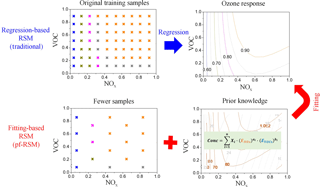Figure 1Schematic plot of comparison between the traditional RSM (regression-based) and the RSM with a polynomial function (denoted as “pf-RSM”, fitting-based).

## 2.2 Development of the pf-RSM

In general, tropospheric O3 and PM2.5 concentrations are contributed to by its sources and sinks through a series of atmospheric processes, such as horizontal or vertical advection and diffusion, gas-phase chemistry, and deposition. The nonlinear behavior in each of these processes contributes to the nonlinearity in the responses of concentrations to precursor emissions. Similar responsive functions can be expected across regions and time. For example, a universal ozone isopleth diagram developed using the empirical kinetic modeling approach of the U.S. Environmental Protection Agency (Gipson et al., 1981) represents the general O3 responsiveness to NO and VOC concentrations. A fitting-based model was developed to simplify the O3 responsiveness to precursor emissions by using a general formulation (Heyes, et al., 1996). The simplified formulation of concentrations to emissions can be easily applied to optimize control strategies (Heyes et al., 1997), which is a great advantage over the regression-based model. Moreover, with the fitting-based RSM, the inclusion of a prior knowledge of pollutant responses to emissions might substantially reduce the case number required to build the RSM (see Fig. 1).

In this study, the prior knowledge of pollutant responses to emissions was characterized as a series of polynomial functions by the previous developed regression-based RSM. The accuracy of the regression-based RSM in representing the nonlinearity in pollutant response to emissions has been examined thoroughly using different methods including cross validation, out-of-sample validation and isopleth validation in previous studies (Xing et al., 2011, 2017a; Wang et al., 2011; Zhao et al., 2015, 2017). The relationship among pollutant responses to emissions followed by the basic chemical functions and physical laws is implicitly represented in the regression-based RSM. In this study, however, we adopted a linear combination of polynomial bases (i.e., 1, x, x2, x3…) to explicitly parameterize the pollutant responses to emissions. The coefficients of the function were estimated by fitting the function with training samples selected brute-force to match with the regression-based RSM prediction (i.e., isopleth validation) and the CMAQ simulations (i.e., out-of-sample validation). The flow scheme of the development of the pf-RSM is displayed in Fig. 2. The structure of the polynomial function to be fitted is expressed as follows:

$\begin{array}{ll}\text{(1)}& & \mathrm{\Delta }\mathrm{Conc}=\sum _{i=\mathrm{1}}^{n}{X}_{i}\cdot {\left({E}_{{\text{NO}}_{x}}\right)}^{{a}_{i}}\cdot {\left({E}_{{\mathrm{SO}}_{\mathrm{2}}}\right)}^{{b}_{i}}\cdot {\left({E}_{{\mathrm{NH}}_{\mathrm{3}}}\right)}^{{c}_{i}}& \cdot {\left({E}_{\mathrm{VOCs}}\right)}^{{d}_{i}}\cdot {\left({E}_{\text{POA}}\right)}^{{e}_{i}},\end{array}$

where ΔConc is the response of O3 and PM2.5 concentrations (i.e., change to the baseline concentration), and the concentration value can be hourly, monthly or annual averages at either a single grid cell or aggregated grids in the target region; ${E}_{{\mathrm{NO}}_{x}}$, ${E}_{{\mathrm{SO}}_{\mathrm{2}}}$, ${E}_{{\mathrm{NH}}_{\mathrm{3}}}$, EVOCs and EPOA are the change ratios of NOx, SO2, NH3, VOCs and POA emissions, respectively, related to the baseline (i.e., baseline = 0); ${a}_{i},{b}_{i},{c}_{i},{d}_{i}$ and ei represent the nonnegative integer powers of ${E}_{{\mathrm{NO}}_{x}}$, ${E}_{{\mathrm{SO}}_{\mathrm{2}}}$, ${E}_{{\mathrm{NH}}_{\mathrm{3}}}$, EVOCs and EPOA, respectively; Xi is the coefficient of the term i. ΔConc is calculated from a polynomial function of five variables (${E}_{{\mathrm{NO}}_{x}}$, ${E}_{{\mathrm{SO}}_{\mathrm{2}}}$, ${E}_{{\mathrm{NH}}_{\mathrm{3}}}$, EVOCs, EPOA). The number of terms (n), coefficients (Xi) and degrees (ai, bi, ci, di, ei) of each term were determined using the following steps.

### 2.2.1 Degree examination

First, the degrees of the five variables were determined individually by fitting the responsive function with a polynomial of a single indeterminate plot (Fig. 3). The PM2.5 responses to the change in each precursor emission estimated using the RSM were fitted by a series of polynomials of a single indeterminate plot with different orders from the first (linear) to the fifth degree, as shown in following functions (similar to Eq. 1):

$\begin{array}{}\text{(2)}& \mathrm{\Delta }\text{Conc}=\sum _{i=\mathrm{1}}^{a}{A}_{i}\cdot {\left({E}_{\text{P}}\right)}^{i},\end{array}$

where ΔConc is the response of O3 and PM2.5 concentrations to changes in individual precursor emissions; EP is the change ratio of one precursor (the subscript “P” can represent NOx, SO2, NH3, VOCs or POA) emission related to the baseline; Ai is the coefficient of term i; and the superscript a is the degree of precursor P, which determined the order of the best fitting polynomials.

Table 1Degree of variables in the polynomial function of response to emission changes.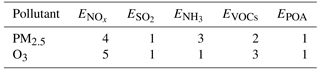* ${E}_{{\mathrm{NO}}_{x}}$, ${E}_{{\mathrm{SO}}_{\mathrm{2}}}$, ${E}_{{\mathrm{NH}}_{\mathrm{3}}}$, EVOCs and EPOA is the change ratio of NOx, SO2, NH3, VOCs and POA emissions, respectively.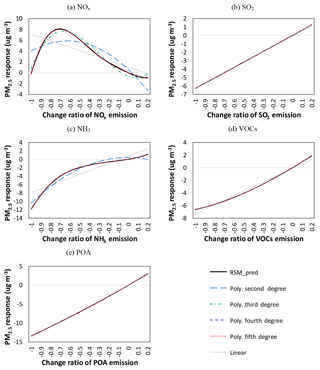Figure 3Fitting the PM2.5 responsive function with a polynomial of a single indeterminate plot.

Figure 3a presents PM2.5 responses to changes in NOx, showing that PM2.5 responses cannot be well fitted with polynomials of the order lower than 3. Better performance is shown in fitting with a fourth-order polynomial (R= 0.999, MeanFE = 0.2) than with a third-order polynomial (R= 0.987, MeanFE = 0.6). Thus the degree of NOx to PM2.5 should be 4. By contrast, PM2.5 responses to changes in SO2 (Fig. 3a) can be well fitted linearly; thus, the degree of SO2 to PM2.5 is 1. The degrees of five precursors to O3 and other pollutants were also examined, and the results are summarized in Table 1. Highly nonlinear responses were found for both O3 and PM2.5 to the NOx, VOC and NH3 emissions. That might be associated with the strong nonlinearity in the atmospheric oxidation reactions and aerosol thermodynamics which are parameterized with the SAPRC99 gas-phase chemistry module and the AERO6 with 2D-VBS module, respectively, in CMAQ used in this study.

### 2.2.2 Term selection

The correlation among variables (i.e., product term) was determined in pairs by fitting the responsive function with a polynomial of a two-indeterminate isopleth, expressed as follows:

$\begin{array}{}\text{(3)}& \mathrm{\Delta }\text{Conc}=\sum _{i=\mathrm{1}}^{b}{B}_{i}\cdot {\left({E}_{{\text{P}}_{\mathrm{1}}}\right)}^{{a}_{i}^{\mathrm{1}}}\cdot {\left({E}_{{\text{P}}_{\mathrm{2}}}\right)}^{{a}_{i}^{\mathrm{2}}},\end{array}$

where ΔConc is the response of O3 and PM2.5 concentrations to changes in individual precursor emissions; ${E}_{{\text{P}}_{\mathrm{1}}}$ and ${E}_{{\text{P}}_{\mathrm{2}}}$ are the change ratios of two precursor (P1 and P2 can represent any two of NOx, SO2, NH3, VOCs or POA) emissions related to the baseline; Bi is the coefficient of product term i; ${a}_{i}^{\mathrm{1}}$ and ${a}_{i}^{\mathrm{2}}$ are the degrees of precursors P1 and P2, respectively; and the superscript b is the number of total interaction terms between P1 and P2 (i.e., ${a}_{i}^{\mathrm{1}}$ multiplied by ${a}_{i}^{\mathrm{2}}\right)$.

The product term ${E}_{{\text{P}}_{\mathrm{1}}}{E}_{{\text{P}}_{\mathrm{2}}}$ represents the interaction between P1 and P2. If no such interaction occurs, the product term ${E}_{{\text{P}}_{\mathrm{1}}}{E}_{{\text{P}}_{\mathrm{2}}}$ is 0. The interaction examination was conducted by comparing predicted responses to joint changes in two precursor emissions between with-interaction Eq. (4) and no-interaction Eq. (5).

$\begin{array}{ll}\text{(4)}& & \mathrm{\Delta }\text{Conc}=\sum _{i=\mathrm{1}}^{a}{A}_{i}\cdot {\left({E}_{{\text{P}}_{\mathrm{1}}}\right)}^{i}+\sum _{j=\mathrm{1}}^{{a}^{\prime }}{A}_{j}{}^{\prime }\cdot {\left({E}_{{\text{P}}_{\mathrm{2}}}\right)}^{j}& +\sum _{i=\mathrm{1}}^{b}{B}_{i}\cdot {\left({E}_{{\text{P}}_{\mathrm{1}}}\right)}^{{a}_{i}^{\mathrm{1}}}\cdot {\left({E}_{{\text{P}}_{\mathrm{2}}}\right)}^{{a}_{i}^{\mathrm{2}}}\\ \text{(5)}& & \mathrm{\Delta }\text{Conc}=\sum _{i=\mathrm{1}}^{a}{A}_{i}\cdot {\left({E}_{{\text{P}}_{\mathrm{1}}}\right)}^{i}+\sum _{j=\mathrm{1}}^{{a}^{\prime }}{A}_{j}{}^{\prime }\cdot {\left({E}_{{\text{P}}_{\mathrm{2}}}\right)}^{j}\end{array}$

If responses calculated using Eq. (5) are equal or approximate to those calculated using Eq. (4), no interactions between P1 and P2 would occur (i.e., the product term ${E}_{{\text{P}}_{\mathrm{1}}}{E}_{{\text{P}}_{\mathrm{2}}}$ is 0). If responses are not equal or approximate to each other, interactions between P1 and P2 cannot be overlooked. However, we wanted to limit the number of terms in the polynomial function; thus, we did not include all interaction terms between P1 and P2 in the function. Instead, we gradually selected interaction terms between P1 and P2 from Eq. (3) until the responses matched with those calculated using Eq. (4).

An example was shown in Supplement Fig. S1, which presents PM2.5 responses to joint changes in NOx and NH3 emissions in July. The PM2.5 response calculated using Eq. (4) (with all interaction terms) was consistent with that estimated using the regression-based RSM. The PM2.5 response calculated using Eq. (5) (with no interaction terms) exhibited a noticeable discrepancy compared with those calculated using Eq. (4) and estimated using the regression-based RSM. With one selected interaction term, the PM2.5 response exhibited a substantial improvement compared with that calculated using Eq. (4), thereby indicating interactions between NOx and NH3 emissions for PM2.5. The results of term selections for both O3 and PM2.5 are summarized in Fig. 4. The interaction terms of NOx and VOCs are included for both pollutants. SO2 and POA did not interact with other species.

### 2.2.3 Sampling optimization

Training samples were generated to fit the polynomial function for each pollutant. To minimize the number of CTM simulations (one simulation scenario represents one training sample), the number of training samples needed to be as small as possible, but greater than the number of terms (i.e., unknown coefficients) in the polynomial function. Our previous study (Xing et al., 2011) suggested that samples generated through uniform methods, such as Latin hypercube sampling (LHS) and a Hammersley quasi-random sequence sample (HSS), could provide even distributions for individual sources. However, additional marginal processing is recommended for its ability to improve the performance of prediction at margins.

Sensitivity analysis of the number and distributions of training samples was conducted in this study. Groups of 20, 30, 40 or 50 training samples were sampled using uniformly distributed HSS. Additional marginal processing was conducted using a power function (n=2) from uniformly distributed HSS on the samples, expressed as follows:

$\begin{array}{}\text{(6)}& \mathrm{\text{TX}}=\left\{\begin{array}{ll}{\left(\frac{X-a}{b-a}\right)}^{\mathrm{2}}×\mathrm{2}×\left(b-a\right)+a,& X\le a+\frac{b-a}{\mathrm{2}}\\ \left[\mathrm{1}-{\left(\frac{b-X}{b-a}\right)}^{\mathrm{2}}×\mathrm{2}\right]×\left(b-a\right)+a,& X>a+\frac{b-a}{\mathrm{2}}\end{array}\right\,\end{array}$

where X is sampled from a uniformly distributed HSS in section [a, b] (in this study we selected [0, 1.2], which denotes that emission changes were from all controlled to a 20 % increase) and TX represents the samples after the marginal processing.

The training samples were predicted using the regression-based RSM and subsequently used to fit the polynomial function for all pollutants. We selected two datasets as out of sample to validate the fitting polynomial function, i.e., jointly controls in five regions (denoted as “OOS100”) and single regional controls (denoted as “OOS15”) (see Table 2). The control matrixes of these two datasets are provided in the Supplement (Table S1). The method of leave-one-out cross validation (LOOCV) was used to examine whether the statistical polynomial regression was overfitting. The definition of LOOCV is to use a single sample from the original datasets as the validation data, and the remaining sample as the training data to build pf-RSM.

The predictive performance of the pf-RSM was evaluated using five statistical indices, namely the mean normalized error (MeanNE), maximal normalized error (MaxNE), mean fractional error (MeanFE), maximal fractional error (MaxFE) and correlation coefficient (R), each calculated as follows:

$\begin{array}{}\text{(7)}& & \text{MeanNE}=\frac{\mathrm{1}}{N}{\sum }_{i=\mathrm{1}}^{N}\frac{\left|{M}_{i}-{O}_{i}\right|}{{O}_{i}}\text{(8)}& & \text{MaxNE}=max\left(\frac{\left|{M}_{i}-{O}_{i}\right|}{{O}_{i}}\right)\text{(9)}& & \text{MeanFE}=\frac{\mathrm{1}}{N}{\sum }_{i=\mathrm{1}}^{N}\frac{\left|{M}_{i}-{O}_{i}\right|}{{M}_{i}+{O}_{i}}×\mathrm{2}\text{(10)}& & \text{MaxFE}=max\left(\frac{\left|{M}_{i}-{O}_{i}\right|}{{M}_{i}+{O}_{i}}×\mathrm{2}\right)\text{(11)}& & R=\sqrt{\frac{\left[{\sum }_{i=\mathrm{1}}^{N}\left({M}_{i}-\stackrel{\mathrm{‾}}{M}\right)\left({O}_{i}-\stackrel{\mathrm{‾}}{O}\right)\right]}{{\sum }_{i=\mathrm{1}}^{N}{\left({M}_{i}-\stackrel{\mathrm{‾}}{M}\right)}^{\mathrm{2}}{\sum }_{i=\mathrm{1}}^{N}{\left({O}_{i}-\stackrel{\mathrm{‾}}{O}\right)}^{\mathrm{2}}}},\end{array}$

where Mi and Oi are the pf-RSM-predicted and CMAQ-simulated value of the ith data in the series, which can be a series of days, grid cells or control cases, and $\stackrel{\mathrm{‾}}{M}$ and $\stackrel{\mathrm{‾}}{O}$ are the average pf-RSM-predicted and CMAQ-simulated value over the series.

## 2.3 Indicators for representing nonlinearity in responses to precursor emissions

In our previous RSM studies, indicators representing the nonlinearity of O3 and PM2.5 responses to precursor emissions have been defined as the peak ratio (PR) for O3 (Xing et al., 2011) and flex ratio (FR) for PM2.5 (Wang et al., 2011), respectively.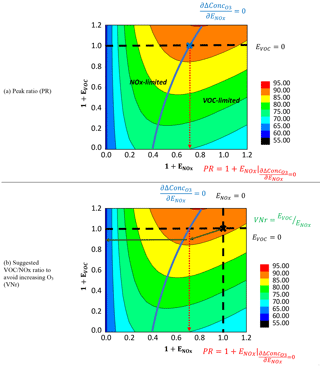Figure 5Definition of peak ratio (PR) and suggested VOC  NOx ratio basing on the 2-D isopleths of O3 sensitivity to NOx and VOC emission changes (an example in Beijing in July).

For O3, the PR is the NOx emissions that produce maximum O3 concentrations under baseline VOC emissions (see in Fig. 5a). A PR lower than 1 (i.e., baseline) indicates that the baseline condition is VOC limited; in all other cases, the baseline condition is NOx limited.

The previous calculations for the PR were performed through a looping procedure in the RSM statistical system, which is not straightforward. One advantage of the pf-RSM is that the PR can be directly calculated from the polynomial function as follows:

$\begin{array}{}\text{(12)}& \text{PR}=\mathrm{1}+{E}_{{\mathrm{NO}}_{x}}{\mathrm{|}}_{\frac{\partial \mathrm{\Delta }{\text{Conc}}_{{\mathrm{O}}_{\mathrm{3}}}}{\partial {E}_{{\mathrm{NO}}_{x}}}=\mathrm{0}}{E}_{{\mathrm{NO}}_{x}}\mathit{ϵ}\left[a,b\right],\end{array}$

where $\frac{\partial \mathrm{\Delta }{\text{Conc}}_{{\mathrm{O}}_{\mathrm{3}}}}{\partial {E}_{{\mathrm{NO}}_{x}}}$ is the first derivation of the ${\text{Conc}}_{{\mathrm{O}}_{\mathrm{3}}}$ response to ${E}_{{\mathrm{NO}}_{x}}$.

In addition, we can further quantify how much simultaneous control of VOC is required to avoid increasing O3 from the NOx controls under VOC-limited conditions (see in Fig. 5b). The suggested VOC controls can be represented as the ratio of VOC to NOx (denoted VNr), which can be calculated as follows:

$\begin{array}{}\text{(13)}& \text{VNr}=X{\mathrm{|}}_{\frac{\partial \mathrm{\Delta }{\text{Conc}}_{{\mathrm{O}}_{\mathrm{3}}}}{\partial {E}_{{\mathrm{NO}}_{x}}}=\mathrm{0}}\phantom{\rule{0.25em}{0ex}}\text{when}\phantom{\rule{0.25em}{0ex}}\text{PR}<\mathrm{1},X={E}_{\text{VOC}}/{E}_{{\mathrm{NO}}_{x}},\end{array}$

where $\frac{\partial \mathrm{\Delta }{\text{Conc}}_{{\mathrm{O}}_{\mathrm{3}}}}{\partial {E}_{{\mathrm{NO}}_{x}}}$ is the first derivation of the ${\text{Conc}}_{{\mathrm{O}}_{\mathrm{3}}}$ response to ${E}_{{\mathrm{NO}}_{x}}$ when ${E}_{\text{VOC}}=X×{E}_{{\mathrm{NO}}_{x}}$.

For PM2.5, here we defined the FR as the NH3 emission ratio at the flex nitrate (or PM2.5) concentrations (i.e., when the second derivation of the function of concentration sensitivities to NH3 emissions is zero) under baseline NOx emissions (see in Fig. 6a). A FR greater than 1 indicates that the baseline condition is NH3  poor (i.e., large sensitivity of PM2.5 to NH3); in all other cases, the baseline condition is NH3 rich (small sensitivity of PM2.5 to NH3). The values of FR also suggest the transition point between two schemes.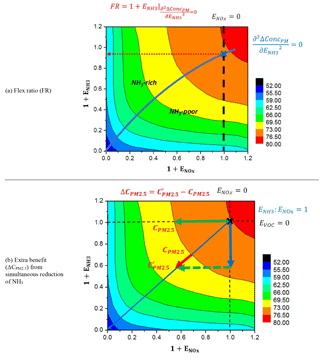Figure 6Definition of flex ratio (FR) and extra benefit from simultaneous reduction of NH3 basing on the 2-D isopleths of PM2.5 sensitivity to NOx and NH3 emission changes (an example in Beijing in July).

Similarly, the FR can be directly calculated from the polynomial function as follows:

$\begin{array}{}\text{(14)}& \text{FR}=\mathrm{1}+{E}_{{\mathrm{NH}}_{\mathrm{3}}}{\mathrm{|}}_{\frac{{\partial }^{\mathrm{2}}\mathrm{\Delta }{\text{Conc}}_{\text{PM}}}{\partial {{E}_{{\mathrm{NH}}_{\mathrm{3}}}}^{\mathrm{2}}}=\mathrm{0}}{E}_{{\mathrm{NH}}_{\mathrm{3}}}\mathit{ϵ}\left[a,b\right],\end{array}$

where $\frac{{\partial }^{\mathrm{2}}\mathrm{\Delta }{\text{Conc}}_{\text{PM}}}{\partial {{E}_{{\mathrm{NH}}_{\mathrm{3}}}}^{\mathrm{2}}}$ is the second derivation of the ConcPM response to ${E}_{{\mathrm{NH}}_{\mathrm{3}}}$.

Further, we can quantify the extra benefit in PM2.5 reductions (denoted as ΔC) from simultaneous reduction of NH3 along with the control of NOx (see in Fig. 6b), which can be calculated as follows:

$\begin{array}{ll}\text{(15)}& & \mathrm{\Delta }C=\left(\frac{\partial \mathrm{\Delta }{\text{Conc}}_{{\text{PM}}_{\mathrm{2.5}}}}{\partial {E}_{{\mathrm{NO}}_{x}}}{\mathrm{|}}_{{E}_{{\mathrm{NH}}_{\mathrm{3}}}={E}_{{\mathrm{NO}}_{x}}}\right)& -\left(\frac{\partial \mathrm{\Delta }{\text{Conc}}_{{\text{PM}}_{\mathrm{2.5}}}}{\partial {E}_{{\mathrm{NO}}_{x}}}{\mathrm{|}}_{{E}_{{\mathrm{NH}}_{\mathrm{3}}}=\mathrm{0}}\right),\end{array}$

where $\frac{\partial \mathrm{\Delta }{\text{Conc}}_{{\text{PM}}_{\mathrm{2.5}}}}{\partial {E}_{{\mathrm{NO}}_{x}}}{\mathrm{|}}_{{E}_{{\mathrm{NH}}_{\mathrm{3}}}={E}_{{\mathrm{NO}}_{x}}}$ is the first derivation of the ${\text{Conc}}_{{\text{PM}}_{\mathrm{2.5}}}$ response to ${E}_{{\mathrm{NO}}_{x}}$ when ${E}_{{\mathrm{NH}}_{\mathrm{3}}}={E}_{{\mathrm{NO}}_{x}}$; $\frac{\partial \mathrm{\Delta }{\text{Conc}}_{{\text{PM}}_{\mathrm{2.5}}}}{\partial {E}_{{\mathrm{NO}}_{x}}}{\mathrm{|}}_{{E}_{{\mathrm{NH}}_{\mathrm{3}}}\phantom{\rule{0.125em}{0ex}}=\phantom{\rule{0.125em}{0ex}}\mathrm{0}}$ is the first derivation of the ${\text{Conc}}_{{\text{PM}}_{\mathrm{2.5}}}$ response to ${E}_{{\mathrm{NO}}_{x}}$ when ${E}_{{\mathrm{NH}}_{\mathrm{3}}}=\mathrm{0}$.

The PR and FR are the results of $\mathrm{1}+{E}_{{\mathrm{NO}}_{x}}$ and $\mathrm{1}+{E}_{{\mathrm{NH}}_{\mathrm{3}}}$, respectively, corresponding to the extreme value point and inflexion point of ${\text{Conc}}_{{\mathrm{O}}_{\mathrm{3}}}$ and ConcPM, respectively, in section [a, b] (i.e., [0, 1.2] in this study). The ratios of VOC to NOx and ΔC were estimated for the five regions in BTH.

3 Results

## 3.1 Sensitivity analysis on training sample number and distribution

Table 3 summarizes the performance of the pf-RSM with different training samples for predicting PM2.5 and O3. For out-of-sample validation (i.e., OOS100 and OOS15), good agreement was observed in all cases. Even with 20 training samples (only five more than the number of terms in the polynomial function), the MeanNE and MeanFE were lower than 3.1 and 1.5 %, respectively, and the MaxNE and MaxFE were lower than 15.1 and 7.0 %, respectively. The R values were greater than 0.8. The performance improved with an increase in training sample number. When 50 training samples were selected, the MeanNE and MeanFE were lower than 1.7 and 0.8 %, respectively, and the MaxNE and MaxFE were lower than 8.7 and 4.2 %, respectively. The R values were greater than 0.94.

Additional marginal processing improved the performance of PM2.5 and O3 prediction by reducing the maximal errors rather than the mean errors. In all cases, the MaxNE and MaxFE in O3 decreased from 12.4 and 5.8 % to 5.5 and 2.7 %, respectively. The MaxNE and MaxFE in PM2.5 slightly decreased from 15.1 and 6.98 % to 15.0 and 6.97 %, respectively.

To meet the criteria of MeanNE within 2 % and MaxNE within 10 % (i.e., uncertainty of pf-RSM), which is comparable to the performance of previous regression-based RSMs, the use of 40 training samples with marginal processing (to improve boundary conditions) is recommended.

Similar results are found in the cross validation (i.e., LOOCV), as the performance in pf-RSM gets better along with the increase in sample numbers. Basically, the statistics of cross validation are in the same order as shown in out-of-sample validations (OOS100 and OOS15), except for the case of 20 training samples with marginal processing (worse performance due to underfitting problem). One interesting finding is that the pf-RSM with marginal processing exhibits worse performance than that with an even sampling method in cross validation. That is because the samples with marginal processing are located closer to margin areas where it is more difficult to predict (Xing et al., 2011). This also implies that the samples with marginal processing have better representation of the variability. Nevertheless, the results of validations suggest the pf-RSM with the current number of samples is not overfitted, and the number of training samples selected in fitting the system is recommended to be 40 training samples with marginal processing.

One kind of visual comparison, i.e., isopleth validation of the pf-RSM with different training samples was conducted, and its details are shown in the Supplement (Fig. S2–S9). The performance of the pf-RSM with less than 40 training samples exhibited a noticeable discrepancy (i.e., spatial pattern of the response under the controls) compared with that of the regression-based RSM. Such discrepancy is caused by the underfitting issue, implying that the number of training samples is not large enough to capture the nonlinearity in the model system. The issue can be addressed by adding more training samples to fit the model. The 40 training samples presented good agreement with the predictions of the regression-based RSM. An improved sampling method is also important for reducing the biases. We can see that additional marginal processing also improved the performance of the pf-RSM.

## 3.2 Application of the polynomial function at different locations and times

First, we applied the pf-RSM in each grid cell in the simulated domain. The base case and 40 controlled scenarios simulated by the CMAQ model (41 training samples in total) were used to fit the function of each grid cell. Two out-of-sample CMAQ cases (i.e., Case 1: moderate control with ${E}_{{\mathrm{NO}}_{x}}$, ${E}_{{\mathrm{SO}}_{\mathrm{2}}}$, ${E}_{{\mathrm{NH}}_{\mathrm{3}}}$, EVOCs and EPOA$=-$49, 45, 20, 64 and 20 % respectively; Case 2: strict control with ${E}_{{\mathrm{NO}}_{x}}$, ${E}_{{\mathrm{SO}}_{\mathrm{2}}}$, ${E}_{{\mathrm{NH}}_{\mathrm{3}}}$, EVOCs and EPOA$=-$76, 79, 81, 83 and 73 %, respectively) were used to validate the performance of the pf-RSM. These two scenarios are selected from the OOS100 to represent two kinds of emission levels, moderate and strict, for the purpose of analyzing the pf-RSM performance under different locations and times. Please note that the validation results might slightly change if we change the scenarios; however, the performance should be similar to the two we presented here (see comparison with the other nine cases shown in Fig. S10).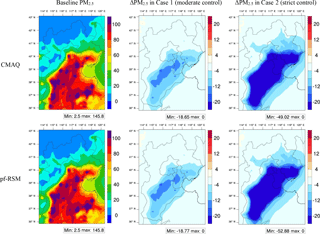Figure 7Spatial distribution of CMAQ-simulated and pf-RSM-predicted PM2.5 in the baseline and PM2.5 responses in two control scenarios (monthly averages in January 2014, unit: µg m−3, ${E}_{{\mathrm{NO}}_{x}}$, ${E}_{{\mathrm{SO}}_{\mathrm{2}}}$, ${E}_{{\mathrm{NH}}_{\mathrm{3}}}$, EVOCs and EPOA in Case 1 and Case 2 are 49, 45, 20, 64, and 20 and 76, 79, 81, 83, and 73 %, respectively).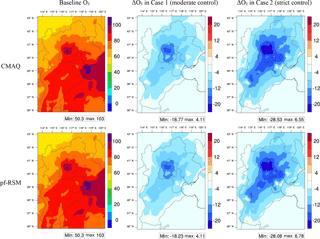Figure 8Spatial distribution of CMAQ-simulated and pf-RSM-predicted O3 in baseline and O3 responses in two control scenarios (monthly averages of daily 1 h maxima O3 in July 2014, unit: ppb, ${E}_{{\mathrm{NO}}_{x}}$, ${E}_{{\mathrm{SO}}_{\mathrm{2}}}$, ${E}_{{\mathrm{NH}}_{\mathrm{3}}}$, EVOCs and EPOA in Case 1 and Case 2 are 49, 45, 20, 64, and 20 and 76, 79, 81, 83, and 73 %, respectively).

Figures 7 and 8 present the spatial distribution of CMAQ-simulated and pf-RSM-predicted PM2.5 and O3 in the baseline and their responses in two control scenarios. PM2.5 predictions by the pf-RSM exhibited the same values in the baseline scenario as those simulated by the CMAQ model because the ΔConc is 0 with no perturbations in emissions; Eq. (1). With the reduction of emissions in the two control cases, the PM2.5 and O3 concentrations were reduced substantially in the CMAQ and pf-RSM predictions. The pf-RSM and CMAQ made very similar predictions for both cases, with normalized errors all within 5.6 % for PM2.5 and 2.0 % for O3 across the domain.

The performance of PM2.5 and O3 prediction in the pf-RSM across grid cells was summarized in Table S2. Larger errors were shown in Case 2 than in Case 1 because of relatively poor performance at the margin areas, where emissions were greatly controlled (Xing et al., 2011). Under moderate control condition (i.e., Case 1), smaller errors were observed in polluted regions for PM2.5 and O3 because of larger denominators (i.e., a high concentration). However, under strict control conditions (i.e., Case 2), larger errors were evident in more polluted regions, particularly for PM2.5, indicating that the biases due to marginal effects were more prevalent in polluted regions.

Second, we applied the pf-RSM to each day in two simulated months (i.e., January and July, 2014). The same 41 training samples and two additional CMAQ cases were used to fit and validate the pf-RSM on each day.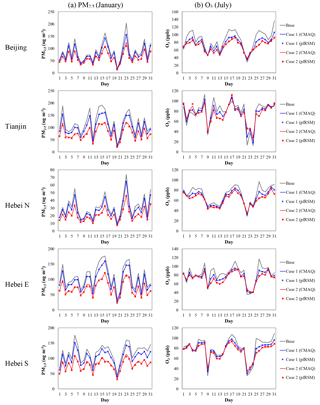Figure 9Daily series of CMAQ-simulated and pf-RSM-predicted daily averaged PM2.5 in January and daily 1 h maxima O3 in July 2014 in the baseline and two control scenarios (${E}_{{\mathrm{NO}}_{x}}$, ${E}_{{\mathrm{SO}}_{\mathrm{2}}}$, ${E}_{{\mathrm{NH}}_{\mathrm{3}}}$, EVOCs and EPOA in Case 1 and Case 2 are 49, 45, 20, 64, and 20 and 76, 79, 81, 83, and 73 %, respectively).

The daily series of the CMAQ-simulated and pf-RSM-predicted 24 h averaged PM2.5 and 1 h maxima O3 in the baseline and two control scenarios are shown in Fig. 9. The day-to-day variability in O3 depends on the budget of O3 source and sink influenced by meteorological variables including actinic flux, temperature, humidity and precipitation, etc. Generally, the pf-RSM-predicted daily PM2.5 and O3 concentrations matched with CMAQ model simulations fairly well, with normalized errors within 12.7 and 6.5 % for PM2.5 and O3, respectively. Substantial reductions in PM2.5 were observed in Case 2, in which strict controls were applied. Noticeable biases were observed on 23 January when PM2.5 levels were high in Beijing and HebeiS. The meteorological conditions will also play an important role in the effectiveness of emission controls. Reductions in O3 were noticeable in both control cases, particularly on days when O3 levels were high. However, increases in O3 were observed on 21–23 July (precipitation event occurred across North China Plain), after the controls were applied and when O3 levels were low. This can be explained by the O3 chemistry scheme being at a strong VOC-limited conditions on days with low O3 levels, resulting in enhanced O3 from NOx controls (Xing et al., 2011). Thus, the emission controls usually become less effective under unfavorable meteorological conditions for O3 production. The pf-RSM also reproduced increases in O3 on those days.

Table 3Performance of PM2.5 and O3 prediction using pf-RSM with different training samples.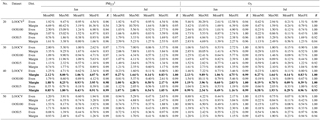1PM2.5 and O3 responses are calculated based on monthly averaged concentrations for averages of urban sites. 2 LOOCV: “leave-one-out cross validation” in which a single sample from the original datasets is used as the validation data, and the remaining samples as the training data to build pf-RSM.

The performance of PM2.5 and O3 prediction in the pf-RSM throughout the simulation period was summarized in Table S3. The MeanNEs for PM2.5 and O3 were within 3.7 and 1.3 %, respectively. Larger errors were evident in Case 2 than in Case 1 because of poor performance at margin areas, where emissions are greatly controlled (Xing et al., 2011). These biases in Case 2 became larger on more polluted days, particularly for PM2.5, suggesting that marginal biases were more evident during polluted period.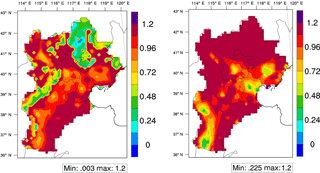Figure 10Spatial distribution of the indicators for PM2.5 (flex ratio, FR) in January and O3 chemistry (peak ratio, PR) in July 2014.

## 3.3 Quantification of nonlinearities in control effectiveness for reducing PM2.5 and O3

The nonlinearity in the pollution response to emissions leads to an either enhanced or reduced effectiveness of emission controls. In previous studies, the concept of NH3-limited/NH3-poor and NOx-/VOC-limited conditions was used widely to demonstrate the influence of NH3 and VOC on effectiveness of NOx controls for reducing PM2.5 and O3, respectively. However, some key questions were not well addressed, such as what percentage of NOx or NH3 is overabundant and what percentage of VOC needs to be reduced simultaneously to avoid increased O3. In this study, the newly developed pf-RSM explicitly represents the response, and the enhanced effectiveness can be easily quantified. The indicators defined in Sect. 2.3 can be used to quantify the nonlinear effectiveness of emission control for reducing PM2.5 and O3. The FR values across grid cells were calculated using Eq. (14) for PM2.5 chemistry in January (Fig. 10a). Most of the study regions exhibited FR values lower than 1, suggesting strong NH3-rich conditions. These results are consistent with those of previous studies (Liu et al., 2010; Wang et al., 2011). Larger FR values (slightly lower than 1.0) were shown in the central and southern regions (i.e., Beijing, Tianjin and HebeiS) than in other regions, suggesting that the PM2.5 concentrations were sensitive to both NOx and NH3 controls, possibly because of the high SO2 and NOx emissions in Beijing, Tianjin and HebeiS (Zhao et al., 2016), which led to the high consumption of NH3 neutralized with H2SO4 and HNO3, as well as high PM2.5 concentrations (Fig. 5).

Table 4 summarized the indicators at urban areas of prefecture-level cities in the five target regions. In both January and July, most of the urban areas (except strong NH3-poor conditions in HebeiN in July) present NH3-rich condition with a FR from 0.75 to 0.95 (Table 4), implying NH3 is sufficiently abundant to neutralize extra nitric acid produced by an additional 5–35 % (i.e., =1  FR-1) of NOx emissions. The result is consistent with our previous study (Wang et al., 2011), which reported that NH3 is sufficiently abundant to neutralize extra nitric acid produced by an additional 25 % of NOx emissions in the North China Plain based on a traditional regression-based RSM study. The extra benefit in PM2.5 reductions from simultaneous reduction of NH3 along with the control of NOx was estimated to be 0.04–0.15 µg m−3 PM2.5 per 1 % reduction of NH3. A larger benefit in PM2.5 reductions by simultaneous reduction of NH3 was found in July when the NH3-rich conditions were not as strong as in January.

Table 4Estimation of indicators that represent the nonlinear control effectiveness for reducing PM2.5 and O3 in the Beijing–Tianjin–Hebei region.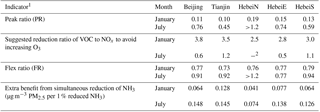1 Indicators are calculated based on monthly averaged concentrations at urban areas of prefecture-level cities in the five target regions. 2 Since the PR is larger than 1.2 in HebeiN, the NOx control will always lead to a reduction in O3.

The PR values for O3 chemistry in July were calculated using Eq. (12), as shown in Fig. 10b. Different PR values were observed in urban and downwind areas. That is consistent with the findings of previous studies (Xing et al., 2011), which used a traditional regression-based RSM and found that the PR changes from 0.8 to 1.2 as the distance from the city center increases. Smaller PRs (0.4–0.8, see Table 4) were evident in urban areas (i.e., megacities such as Beijing, Tianjin, Shijiazhuang and Tangshan), where NOx emissions are saturated, resulting in strong VOC-limited conditions. Our results are consistent with the observational studies that use an indicator to identify the O3 chemistry. For example, Liu et al. (2016) studied the ratios of HCHO over NO2 from the satellite retrievals and found that local ozone production in urban Beijing is VOC limited when there are no substantial changes in NOx emission in 2015. Chou et al. (2009) found that the Beijing urban area was a VOC-limited region based on the observation of NO, NOx and NOy at the Peking University site from 15 August to 11 September in 2006. Jin and Holloway (2015) calculated the ratio of HCHO to NO2 from the OMI instrument aboard the Aura satellite and found the O3 production is more likely to be VOC limited over urban areas and NOx limited over rural and remote areas in China from 2005 to 2013.

The PR values calculated in this study also indicate that the control of NOx (with less than 20–60 % reduction, = 1  PR) could result in an increase in O3; however, O3 would decrease with substantial control of NOx (with greater than 20–60 % reduction). To avoid increasing O3 during the transition from VOC-limited to NOx-limited conditions, a simultaneous VOC reduction by 0.5–1.2 times the rate of NOx reduction is recommended. Stronger VOC-limited conditions are found in January, while O3 concentration is considerably lower than in July. However, the strong VOC-limited conditions in January will also lead to a considerable disbenefit of NOx reduction for PM2.5 controls (see the isopleth plot of PM2.5 response to NOx and NH3 emission changes in Fig. S6, also found in Zhao et al., 2017) because the enhanced atmospheric oxidation ability from reducing NOx under VOC-limited conditions will facilitate the formation of secondary aerosols. Therefore simultaneous VOC reduction can help avoid such increase of PM2.5 associated with NOx controls under strong VOC-limited condition in January. Notably, the O3 discussed in this paper refers to the monthly averages of daily 1 h maximum values. The PR values varied considerably between the clean and polluted days, suggesting mostly NOx-limited conditions during polluted periods, which are usually subject to a more severe O3 burden (Xing et al., 2011). Nevertheless, the control of NOx emissions is critical for reducing regional O3 and PM2.5; however, it is recommended to simultaneously reduce VOC and NH3 emissions along with NOx reduction to avoid the risk of increasing O3 and gain extra benefit in PM2.5 reduction.

4 Summary and conclusion

Quantification of the effectiveness of air pollution controls by emission mitigation needs an accurate representation of the nonlinear responses of ambient O3 and PM2.5 concentrations to precursor emission changes. To address this challenge, this study proposed a new method by fitting multiple simulations of a CTM with a set of polynomial functions, called “pf-RSM”. The pf-RSM method was successfully applied in a study of the BTH region in China. The pf-RSM method characterizes the nonlinearity in the air quality response to emission changes. In the polynomial functions developed in this study, high degrees were found for the responses to the emissions of NOx, VOC and NH3, which exhibit stronger nonlinear behavior than SO2 and POA. The interaction terms of NOx and VOC are included for both PM2.5 and O3, indicating that atmospheric oxidations play a significant role in the nonlinearity of air quality responses. The interaction term of NOx and NH3 emissions is also considered for PM2.5, suggesting nonlinearity in nitrate formation and aerosol thermodynamics.

After the application of a prior knowledge of the pollutant responsiveness to emissions in the RSM system, the cases required for single regional pf-RSM development were substantially decreased to 40 samples, compared with the previous requirement of over 100 samples, implying that the fitting-based RSM (i.e., pf-RSM) is 3 times faster than previous regression-based RSMs (i.e., the number of CTM simulations needed in pf-RSM is 60 % less than that required by previous regression-based RSMs). The pf-RSM system in this study operates rapidly, and thus can quickly generate responses with high spatial and temporal resolutions, thereby further facilitating cost-benefit optimization and enabling further assessment studies to be conducted (e.g., air pollution control, cost-benefit and attainment assessment ABaCAS system described by Xing et al., 2017b). The polynomial functions developed in this study have been successfully applied in all grid cells across the simulated domain and all days across the simulated periods for both January and July, indicating that the combination of terms selected in this study is spatially and temporally independent as it mainly depends on the nonlinearity in the atmospheric processes. It means that only the coefficients of terms need to be fitted with training samples in another case (Step 3 in Fig. 2), as seen in Table S4, which provides the coefficients of 15 terms for PM2.5 and O3 in the BTH region. The degrees and selected terms (Steps 1–2 in Fig. 2) do not need to be recalculated unless there have been significant updates in the chemistry mechanism in the CTM. However, it might need to be further confirmed by more applications in other regions outside BTH and for a year-long analysis to better represent the seasonality.

Based on the pf-RSM, a series of indicators were calculated from the polynomial function to represent the nonlinearity in control effectiveness for reducing PM2.5 and O3, including peak ratio (i.e., PR), suggested VOC  NOx ratio to avoid increasing O3 (i.e., the ratio of VOC to NOx), flex ratio (i.e., FR) and the extra benefit from simultaneous reduction of NH3 (µg m−3 PM2.5 per 1 % reduced NH3). We found strong VOC-limited conditions and NH3-rich conditions for O3 and PM2.5, respectively, in most urban areas of BTH. Results suggest that the NOx emission reduction rate needs to be greater than 20–60 % to pass the transition from VOC limited to NOx limited, and a simultaneous VOC reduction by 0.5–1.2 times the rate of NOx reduction is recommended to avoid increasing O3 during the transition in July. Along with the control of NOx, the simultaneous reduction of NH3 can provide a considerable benefit in PM2.5 reduction by 0.04–0.15 µg m−3 per 1 % reduction of NH3. Our results demonstrate the importance of simultaneous reductions of VOC and NH3 emissions to enhance the effectiveness of air pollution controls by NOx emission reductions in the Beijing–Tianjin–Hebei region in China.

Data availability

Model outputs and pf-RSM code package are available upon request from the corresponding author.

Supplement

Acknowledgements

This work was supported in part by the National Key R and D program of China (2016YFC0207601), National Research Program for Key Issues in Air Pollution Control (DQGG0301), National Science Foundation of China (21625701 and 21521064) and Shanghai Environmental Protection Bureau (2016-12). This work was completed on the “Explorer 100” cluster system of Tsinghua National Laboratory for Information Science and Technology. The authors also acknowledge the contributions of Xiaoyue Niu, Qi Li, Kui Hua and Nayang Shan from the Center for Statistical Science at Tsinghua University.

Edited by: Bryan N. Duncan
Reviewed by: two anonymous referees

References

Appel, K. W., Pouliot, G. A., Simon, H., Sarwar, G., Pye, H. O. T., Napelenok, S. L., Akhtar, F., and Roselle, S. J.: Evaluation of dust and trace metal estimates from the Community Multiscale Air Quality (CMAQ) model version 5.0, Geosci. Model Dev., 6, 883–899, https://doi.org/10.5194/gmd-6-883-2013, 2013.

Carter, W. P.: The SAPRC-99 chemical mechanism and updated VOC reactivity scales, California Air Resources Board, 2003.

Cohan, D. S., Hakami, A., Hu, Y., and Russell, A. G.: Nonlinear response of ozone to emissions: source apportionment and sensitivity analysis, Environ. Sci. Technol., 39, 6739–6748, 2005.

Cohen, A. J., Brauer, M., Burnett, R., Anderson, H. R., Frostad, J., Estep, K., Balakrishnan, K., Brunekreef, B., Dandona, L., Dandona, R., and Feigin, V.: Estimates and 25-year trends of the global burden of disease attributable to ambient air pollution: an analysis of data from the Global Burden of Diseases Study 2015, The Lancet, 389, 1907–1918, 2017.

Chou, C. C.-K., Tsai, C.-Y., Shiu, C.-J., Liu, S. C., and Zhu, T.: Measurement of NOy during Campaign of Air Quality Research in Beijing 2006 (CAREBeijing-2006): Implications for the ozone production efficiency of NOx, J. Geophys. Res., 114, D00G01, doi:10.1029/2008JD010446, 2009.

Dunker, A. M., Yarwood, G., Ortmann, J. P., and Wilson, G. M.: Comparison of source apportionment and source sensitivity of ozone in a three-dimensional air quality model, Environ. Sci. Tech., 36, 2953–2964, 2002.

Foley, K. M., Napelenok, S. L., Jang, C., Phillips, S., Hubbell, B. J., and Fulcher, C. M.: Two reduced form air quality modeling techniques for rapidly calculating pollutant mitigation potential across many sources, locations and precursor emission types, Atmos. Environ., 98, 283–289, 2014.

Forouzanfar, M. H., Alexander, L., Anderson, H. R., Bachman, V. F., Biryukov, S., Brauer, M., Burnett, R., Casey, D., Coates, M. M., Cohen, A., and Delwiche, K.: Global, regional, and national comparative risk assessment of 79 behavioural, environmental and occupational, and metabolic risks or clusters of risks in 188 countries, 1990–2013: a systematic analysis for the Global Burden of Disease Study 2013, The Lancet, 386, 2287–2323, 2015.

Fu, X., Wang, S., Xing, J., Zhang, X., Wang, T., and Hao, J.: Increasing Ammonia Concentrations Reduce the Effectiveness of Particle Pollution Control Achieved via SO2 and NOx Emissions Reduction in East China, Environ. Sci. Tech. Let., 4, 221–227, https://doi.org/10.1021/acs.estlett.7b00143, 2017.

GBD-MAPS project report, New Study: Air pollution from coal a major source of health burden in China, available at: https://www.healtheffects.org/system/files/HEI-GBD-MAPS-China-Press-Release.pdf, 2016.

Gipson, G. L., Freas, W. P., Kelly, R. F., and Meyer, E. L.: Guideline for use of city-specific EKMA in preparing ozone SIPs. EPA-450/4-80-027, US Environmental Protection Agency, Research Triangle Park, North Carolina, USA, 1981.

Hakami, A., Odman, M. T., and Russell, A. G.: High-order, direct sensitivity analysis of multidimensional air quality models, Environ. Sci. Technol., 37, 2442–2452, 2003.

Heyes, C., Schöpp, W., Amann, M., and Unger, S.: A Reduced-Form Model to Predict Long-Term Ozone Concentrations in Europe, Interim Report WP-96-12, 1996.

Heyes, C., Schöpp, W., Amann, M., Bertok, I., Cofala, J., Gyarfas, F., Klimont, Z., Makowski, M., and Shibayev, S.: A model for optimizing strategies for controlling ground-level ozone in Europe, Interim Report IR-97-002, 1997.

Jin, X. and Holloway, T.: Spatial and temporal variability of ozone sensitivity over China observed from the Ozone Monitoring Instrument, J. Geophys. Res.-Atmos., 120, 7229–7246, 2015.

Kwok, R. H. F., Napelenok, S. L., and Baker, K. R.: Implementation and evaluation of PM2.5 source contribution analysis in a photochemical model, Atmos. Environ., 80, 398–407, 2013.

Kwok, R. H. F., Baker, K. R., Napelenok, S. L., and Tonnesen, G. S.: Photochemical grid model implementation and application of VOC, NOx, and O3 source apportionment, Geosci. Model Dev., 8, 99–114, https://doi.org/10.5194/gmd-8-99-2015, 2015.

Li, J., Lu, K., Lv, W., Li, J., Zhong, L., Ou, Y., Chen, D., Huang, X., and Zhang, Y.: Fast increasing of surface ozone concentrations in Pearl River Delta characterized by a regional air quality monitoring network during 2006–2011, J. Environ. Sci., 26, 23–36, 2014.

Liu, H., Liu, C., Xie, Z., Li, Y., Huang, X., Wang, S., Xu, J., and Xie, P.: A paradox for air pollution controlling in China revealed by “APEC Blue” and “Parade Blue”, Scientific Reports, 6, 34408, https://doi.org/10.1038/srep34408, 2016.

Liu, X.-H., Zhang, Y., Xing, J., Zhang, Q., Streets, D. G., Jang, C. J., Wang, W.-X., and Hao, J.-M.: Understanding of Regional Air Pollution over China using CMAQ – Part II. Process Analysis and Ozone Sensitivity to Precursor Emissions, Atmos. Environ., 44, 3719–3727, 2010.

Liu, F., Zhang, Q., Zheng, B., Tong, D., Yan, L., Zheng, Y. and He, K.: Recent reduction in NOx emissions over China: synthesis of satellite observations and emission inventories, Environ. Res. Lett., 11, 114002, https://doi.org/10.1088/1748-9326/11/11/114002, 2016.

Myhre, G., Shindell, D., Bréon, F.M., Collins, W., Fuglestvedt, J., Huang, J., Koch, D., Lamarque, J. F., Lee, D., Mendoza, B., and Nakajima, T.: Anthropogenic and Natural Radiative Forcing, in: Climate Change 2013: The Physical Science Basis, Contribution of Working Group I to the Fifth Assessment Report of the Intergovernmental Panel on Climate Change, edited by: Stocker T. F. et al., Cambridge Univ. Press, 659–740, 2013.

Santner, T. J., Williams, B. J., and Notz, W.: The Design and Analysis of Computer Experiments, Springer Verlag, New York, 2003.

Seinfeld, J. H. and Pandis, S. N.: Atmospheric chemistry and physics: From air pollution to climate change, John Wiley and Sons, Inc., 241 pp, 2006.

Sillman, S.: The use of NOy, H2O2, and HNO3 as indicators for ozone-NOx-hydrocarbon sensitivity in urban locations, J. Geophys. Res., 100, 4175–4188, 1995.

Tonnesen, G. S. and Dennis, R. L.: Analysis of radical propagation efficiency to assess ozone sensitivity to hydrocarbons and NOx 1, Local indicators of instantaneous odd oxygen production sensitivity, J. Geophys. Res., 105, 9213–9225, 2000.

Tsimpidi, A. P., Karydis, V. A., and Pandis, S. N.: Response of Fine Particulate Matter to Emission Changes of Oxides of Nitrogen and Anthropogenic Volatile Organic Compounds in the Eastern United States, J. Air Waste. Manage. Assoc., 58, 1463–1473, doi:10.3155/1047-3289.58.11.1463, 2008.

Wagstrom, K. M., Pandis, S. N., Yarwood, G., Wilson, G. M., and Morris, R. E.: Development and application of a computationally efficient particulate matter apportionment algorithm in a three-dimensional chemical transport model, Atmos. Environ., 42, 5650–5659, 2008.

Wang, S. X., Xing, J., Jang, C., Zhu, Y., Fu, J. S., and Hao, J.: Impact assessment of ammonia emissions on inorganic aerosols in east China using response surface modeling technique, Environ. Sci. Technol., 45, 9293–9300, 2011.

Xing, J., Wang, S. X., Jang, C., Zhu, Y., and Hao, J. M.: Nonlinear response of ozone to precursor emission changes in China: a modeling study using response surface methodology, Atmos. Chem. Phys., 11, 5027–5044, https://doi.org/10.5194/acp-11-5027-2011, 2011.

Xing, J., Wang, S., Zhao, B., Wu, W., Ding, D., Jang, C., Zhu, Y., Chang, X., Wang, J., Zhang, F., and Hao, J.: Quantifying Nonlinear Multiregional Contributions to Ozone and Fine Particles Using an Updated Response Surface Modeling Technique, Environ. Sci. Tech., 51, 11788–11798, 2017a.

Xing, J., Wang, S., Jang, C., Zhu, Y., Zhao, B., Ding, D., Wang, J., Zhao, L., Xie, H., and Hao, J.: ABaCAS: an overview of the air pollution control cost-benefit and attainment assessment system and its application in China, The Magazine for Environmental Managers – Air & Waste Management Association, April, 2017b.

Ye, L., Wang, X., Fan, S., Chen, W., Chang, M., Zhou, S., Wu, Z., and Fan, Q.: Photochemical indicators of ozone sensitivity: application in the Pearl River Delta, China, Front. Env. Sci. Eng., 10, 15, https://doi.org/10.1007/s11783-016-0887-1, 2016.

Zhang, Y., Wen, X.-Y., Wang, K., Vijayaraghavan, K., and Jacobson, M. Z.: Probing into Regional O3 and PM Pollution in the U.S., Part II, An Examination of Formation Mechanisms through a Process Analysis Technique and Sensitivity Study, J. Geophys. Res., 114, D22305, doi:10.1029/2009JD011900, 2009.

Zhao, B., Wang, S. X., Liu, H., Xu, J. Y., Fu, K., Klimont, Z., Hao, J. M., He, K. B., Cofala, J., and Amann, M.: NOx emissions in China: historical trends and future perspectives, Atmos. Chem. Phys., 13, 9869–9897, https://doi.org/10.5194/acp-13-9869-2013, 2013.

Zhao, B., Wang, S. X., Xing, J., Fu, K., Fu, J. S., Jang, C., Zhu, Y., Dong, X. Y., Gao, Y., Wu, W. J., Wang, J. D., and Hao, J. M.: Assessing the nonlinear response of fine particles to precursor emissions: development and application of an extended response surface modeling technique v1.0, Geosci. Model Dev., 8, 115–128, https://doi.org/10.5194/gmd-8-115-2015, 2015a.

Zhao, B., Wang, S., Donahue, N. M., Chuang, W., Hildebrandt Ruiz, L., Ng, N. L., Wang, Y., and Hao, J.: Evaluation of one-dimensional and two-dimensional volatility basis sets in simulating the aging of secondary organic aerosols with smog-chamber experiments, Environ. Sci. Technol., 49, 2245–2254, doi:10.1021/es5048914, 2015b.

Zhao, B., Wang, S., Donahue, N. M., Jathar, S.H., Huang, X., Wu, W., Hao, J., and Robinson, A. L.: Quantifying the effect of organic aerosol aging and intermediate-volatility emissions on regional-scale aerosol pollution in China, Sci. Rep., 6, 28815, doi:10.1038/srep28815, 2016.

Zhao, B., Wu, W., Wang, S., Xing, J., Chang, X., Liou, K.-N., Jiang, J. H., Gu, Y., Jang, C., Fu, J. S., Zhu, Y., Wang, J., Lin, Y., and Hao, J.: A modeling study of the nonlinear response of fine particles to air pollutant emissions in the Beijing-Tianjin-Hebei region, Atmos. Chem. Phys., 17, 12031–12050, https://doi.org/10.5194/acp-17-12031-2017, 2017.